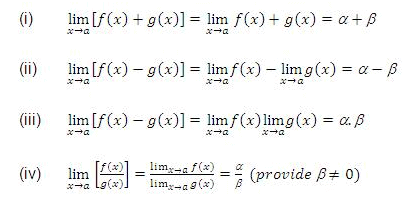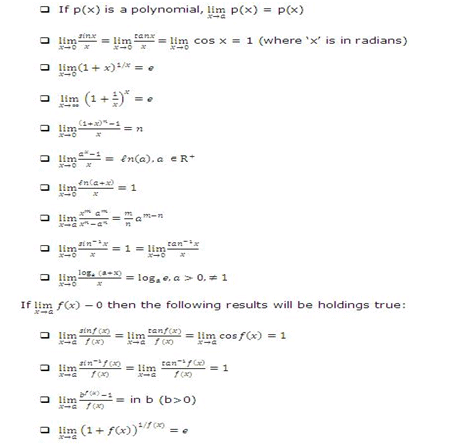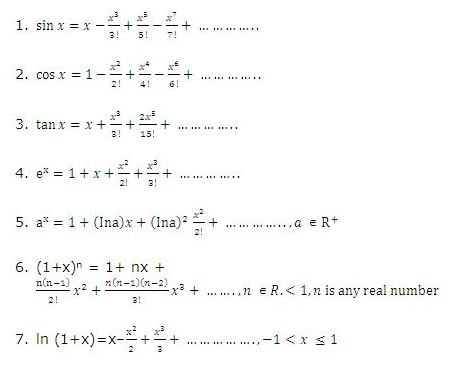# Revision Notes - Limits Notes | Study Mock Test Series for JEE Main & Advanced 2022 - JEE

## JEE: Revision Notes - Limits Notes | Study Mock Test Series for JEE Main & Advanced 2022 - JEE

The document Revision Notes - Limits Notes | Study Mock Test Series for JEE Main & Advanced 2022 - JEE is a part of the JEE Course Mock Test Series for JEE Main & Advanced 2022.
All you need of JEE at this link: JEE
• Limit of a function may be a finite or an infinite number.
• If limx→a f(x) = ∞, it just implies that the function f(x) tends to assume extremely large positive values in the vicinity of x = a i.e. limx→0 1/|x|= ∞.
• A function is said to be indeterminate at any point if it acquires one of the following values at that particular point:
0/0, 0 × ∞, ∞/∞, ∞-∞, 00, 1, ∞0.
• The 0/0 form is the standard indeterminate form.
• The point ‘∞’ cannot be plotted on the paper. It is just a symbol and not a number.

Infinity (∞) does not obey the laws of elementary algebra.
1. ∞ + ∞ = ∞
2. ∞ x ∞ = ∞
3. (a/∞) = 0, if a is finite
4. (a/0) is not defined if a ≠ 0.
5. ab = 0 iff either a = 0 or b = 0 and both‘a’ and ‘b’ are finite.
In case of limits, it is important to note that the function cannot be manipulated and cancelled as in usual algebra.
For example: (x2-a2)/(x-a) = (x+a)(x-a)/((x-a)) = (x+a)
This can be done in general, but in limits this is not possible until and unless (x-a) ≠ 0 or x ≠ a.

• The limit may exist at a point x = a even if the function is not defined at that point.
• If a function f is defined at a point ‘a’ i.e. f(a) exists even then it is not necessary that the limit at ‘a’ should exist. Moreover, even if the limit exists it need not be equal to f(a).
• Fundamental Results on Limits:
Suppose limx→a f(x) = α and limx→a g(x)=β then we can define the following rules:• The above rules are applicable only when both the limits i.e. lim f(x) and g(x) exist separately. In addition to above rules, we have two more rules:
ü limx→a kf(x)= k limx→a f(x), where k is a constant
ü limx→a f [g(x)] = f [limx→a g(x)] = f(m), provided f is continuous at g(x) = m.
• Some standard limits which should be remembered include:Following are some of the frequently used series expansions:• The term infinite limit means that when x tends to a particular value 'a', then the limit of the function tends to infinity i.e.limx→2 f(x) = ∞.
• In questions which involve the evaluation if limit at infinity, the function f(x) should first be changed to g(1/x) and then we can evaluate the value at ∞.
• If while calculating limits, infinite limit is encountered i.e. a zero is obtained in the denominator as x→a, then there can be two cases:
1. The term (x-a) gets cancelled from the numerator and denominator both.
2. If it does not get cancelled, then the value of the limit is put as infinity.
3. Such limits are termed as improper limits i.e. limx→∞ 1/x2 =∞.
• Let f(x), g(x) and h(x) be there real numbers having a common domain D such that h (x) ≤ f(x) ≤ g(x) ∀ x ∈ D. If limx→a h(x) =limx→a g(x)= l, then limx→a f(x) = l. This is known as Sandwich Theorem.
• Let f(x) and g(x) be functions differentiable in the neighbourhood of the point a, except may be at the point a itself. If  limx→a f(x) = 0 = limx→a g(x) or limx→af(x)= ∞ = limx→ag(x), then
limx→a f(x)/g(x) = limx→a f' (x)/g(x) = limx→a f' (x)/g'(x)    provided that the limit on the right either exists as a finite number or is ± ∞ .
The concept of limits and continuity is quite interrelated. Limits form the base for continuity.
The document Revision Notes - Limits Notes | Study Mock Test Series for JEE Main & Advanced 2022 - JEE is a part of the JEE Course Mock Test Series for JEE Main & Advanced 2022.
All you need of JEE at this link: JEEUse Code STAYHOME200 and get INR 200 additional OFF

## Mock Test Series for JEE Main & Advanced 2022

2 videos|258 docs|160 tests

Track your progress, build streaks, highlight & save important lessons and more!

,

,

,

,

,

,

,

,

,

,

,

,

,

,

,

,

,

,

,

,

,

;